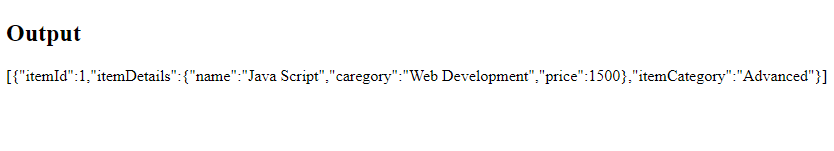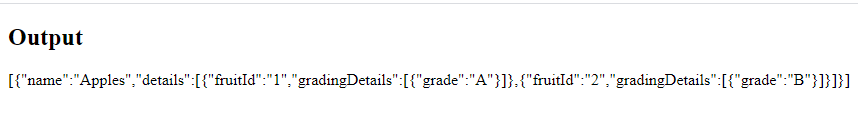# How to filter nested objects in JavaScript ?

• Difficulty Level : Medium
• Last Updated : 05 Nov, 2020

The filter() method creates a new array with all elements that pass the test implemented by the provided function.

Approach 1: This approach uses filter() method to filter the nested object in JavaScript.

Example:

## HTML

 ` ` `<``html``> ` ` `  `<``body``> ` `    ``<``h2``>Output ` `    ``<``p` `id``=``"Output"``> ` ` `  `    ``<``script``> ` `        ``var nestedObject = [ ` `            ``{ ` `                ``itemId: 1, ` `                ``itemDetails: { ` `                    ``name: "C", ` `                    ``caregory: "Programming Language", ` `                    ``price: 500, ` `                ``}, ` `                ``itemCategory: "Basic", ` `            ``}, ` `            ``{ ` `                ``itemId: 2, ` `                ``itemDetails: { ` `                    ``name: "C++", ` `                    ``caregory: "Programming Language", ` `                    ``price: 700, ` `                ``}, ` `                ``itemCategory: "Beginner", ` `            ``}, ` `            ``{ ` `                ``itemId: 1, ` `                ``itemDetails: { ` `                    ``name: "Java Script", ` `                    ``caregory: "Web Development", ` `                    ``price: 1500, ` `                ``}, ` `                ``itemCategory: "Advanced", ` `            ``}] ` `        ``let itemNames = nestedObject.filter( ` `            ``eachObj => eachObj.itemDetails.price === 1500); ` ` `  `        ``document.getElementById("Output").innerHTML ` `            ``= JSON.stringify(itemNames); ` `    `` ` ` ` ` `  ` `

Output:In the above example, only “JavaScript” is the name of the course with price “1500”.

Approach 2: This approach uses some() method to filter the nested objects. The some() method tests whether at least one element in the array passes the test implemented by the provided function. It returns a Boolean value.

Example:

## HTML

 ` ` `<``html``> ` ` `  `<``body``> ` `    ``<``h2``>Output ` `    ``<``p` `id``=``"Output"``> ` ` `  `    ``<``script``> ` `        ``const fruitData = [ ` `            ``{ ` `                ``name: "Apples", ` `                ``details: [ ` `                    ``{ ` `                        ``fruitId: '1', ` `                        ``gradingDetails: [{ grade: 'A' }] ` `                    ``}, ` `                    ``{ ` `                        ``fruitId: '2', ` `                        ``gradingDetails: [{ grade: 'B' }] ` `                    ``} ` `                ``] ` `            ``}, ` `            ``{ ` `                ``name: "Oranges", ` `                ``details: [ ` `                    ``{ ` `                        ``fruitId: '3', ` `                        ``gradingDetails: [{ grade: 'B' }] ` `                    ``}, ` `                    ``{ ` `                        ``fruitId: '4', ` `                        ``gradingDetails: [{ grade: 'D' }] ` `                    ``} ` `                ``] ` `            ``}, ` `        ``]; ` ` `  `        ``let output = fruitData.filter(eachVal => { ` `            ``let opt = eachVal.details.some(( ` `                ``{ gradingDetails }) => gradingDetails ` `                ``.some(({ grade }) => grade === 'A')); ` `            ``return opt; ` `        ``}) ` `        ``console.log(output); ` `        ``document.getElementById("Output").innerHTML = ` `            ``JSON.stringify(output); ` `    `` ` ` ` ` `  ` `

Output:In the above example, the fruitId is 1 for “Apples”and grade is “A”. But for Oranges neither of them satisfies the option.

My Personal Notes arrow_drop_up
Recommended Articles
Page :